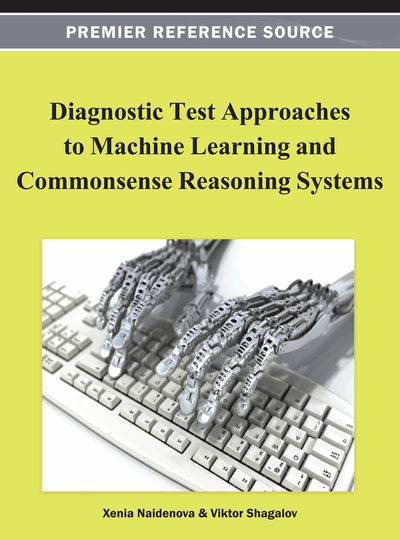# Logical Inference and Defeasible Reasoning in N-tuple Algebra

Boris Kulik (Institute of Problems in Mechanical Engineering, Russian Academy of Sciences (RAS), Russia), Alexander Fridman (Institute for Informatics and Mathematical Modelling, Russia) and Alexander Zuenko (Institute for Informatics and Mathematical Modelling, Russia)
DOI: 10.4018/978-1-4666-1900-5.ch005

## Abstract

This chapter examines the usage potential of n-tuple algebra (NTA) developed by the authors as a theoretical generalization of structures and methods applied in intelligence systems. NTA supports formalization of a wide set of logical problems (abductive and modified conclusions, modelling graphs, semantic networks, expert rules, etc.). This chapter mostly focuses on implementation of logical inference and defeasible reasoning by means of NTA. Logical inference procedures in NTA can include, besides the known logical calculus methods, new algebraic methods for checking correctness of a consequence or for finding corollaries to a given axiom system. Inference methods consider (above feasibility of certain substitutions) inner structure of knowledge to be processed, thus providing faster solving of standard logical analysis tasks. Matrix properties of NTA objects allow decreasing the complexity of intellectual procedures. As for making databases more intelligent, NTA can be considered as an extension of relational algebra to knowledge processing.
Chapter Preview
Top

## Introduction

Many techniques and theories in semantic and logical analysis of information use the concept of “relation.”

Theoretical basics of mathematical logic are expressed in formal language of predicate calculus (Mendelson, 1997). The formulas of mathematical logic can be expressed as tables with sets of satisfiable substitutions, i.e. relations as well.

There are different descriptive languages in artificial intelligence. Nevertheless, as a rule, we can transform examples introduced in publications in order to illustrate different methods and approaches to structures of the type N(E1, E2,…), where N is the name of a relation or a predicate, and E1, E2,… are names of objects belonging to certain totalities of property (attribute) values (Russel and Norvig, 2003). Operations on such structures correspond completely to those of algebra of sets.

Despite wide usage of the concept of relation in mathematics and artificial intelligence, no general theory of relations has yet been developed. The term “theory of relations” is commonly used either for theory of binary relations dealing with graphs, semantic networks, etc. or for theory of n-ary relations based on relational algebra (RA) (Codd, 1970, 1972).

In particular, binary relations are used in formal concept analysis (Ganter and Wille, 1999; Kuznetsov and Schmidt, 2007) and in Description Logic (Baader, 2003), but these logics are not applicable to logical analysis of n-ary relations when n > 2. As for databases based on relational algebra, logical analysis there is possible for some special cases (for instance, Armstrong's axioms which are true for functionally dependent attributes only) or by using recursion in deductive database management systems (DBMSs) (Ceri et al., 1990). Though even in such systems, analytical capabilities of logical analysis are weaker than in predicate calculus.

In any case, these theories accept the classical mathematical definition of a relation through Cartesian product. If D is a Cartesian product of n different or equal sets, then an n-ary relation R is a certain subset of elementary n-tuples contained in D.

Such a definition of an n-ary relation allows treating relations as ordinary sets if they are defined on the same Cartesian product D. However, this feature is no longer valid in totalities of relations defined on various Cartesian products since it is impossible to determine operations of union and intersection for them. Besides, it is desirable to implement operations of composition and join defined in relational algebra and theory of binary relations, but having no equivalent operations in algebra of sets. In other words, algebra of sets does not provide means to process arbitrary relations. In order to take this possibility, we need to introduce some additional operations besides the classical operations of algebra of sets.

Moreover, computer-aided information processing based on applying the classical definition of the relation interpreted as a set of elementary n-tuples, often leads to redundancy caused by multiple replications of the same elements in memory.

As an example, let us consider a relation which reflects the fact that a professor Smith teaches subjects Mathematics, Logic, and Physics: {(Smith, Mathematics), (Smith, Logic), (Smith, Physics)}. This relation can be compacted as a Cartesian product {Smith}×{Mathematics, Logic, Physics}. Obviously, not every relation can be represented as a single Cartesian product composed of non-elementary sets. For instance, the following relation cannot be expressed this way:

## Complete Chapter List

Search this Book:
Reset